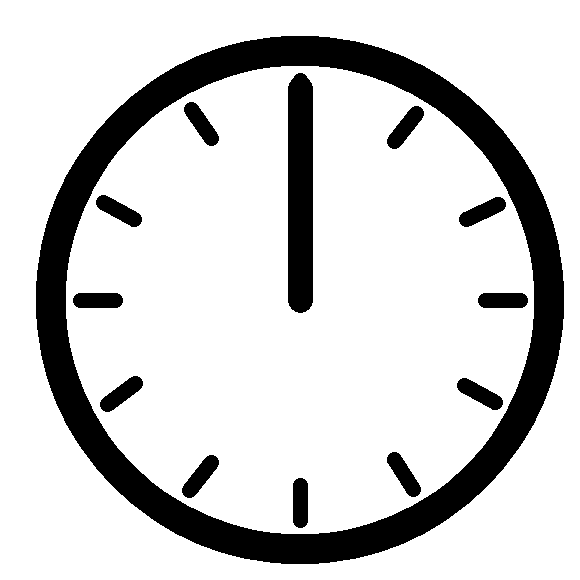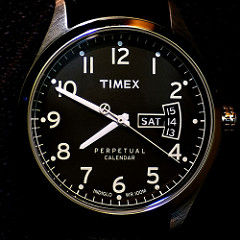﻿ Aligned Clock Hands# Aligned Clock HandsA short little puzzle this week building off an interview question I read on the internet. The original question was this:

At what times of the day do the hour and minute hands of an analog clock perfectly align?

At first glance this might seem like a trivial question, then you realize that the hour hand moves smoothly and continuously around the dial (albeit at a slower pace than the minute hand), and does not snap to each quantized hour position on each hour. This complicates things a little, but not too much. (The answers are not 1:05, 2:10, 3:15 …)It’s pretty clear that the hands both align when it’s exactly midnight (and midday). When is the next time? It’s not 1:05, but a little bit past because, by the time the minute hand is also at the 1 o’clock position, the hour hand will have progressed on slightly. The minute hand spins around the dial twelve times as fast as the hour hand (it completes one revolution in an hour whilst the hour hand moves one hour, which is 1/12th of the clock face).

In T hours, the minute hand completes T revolutions. In the same amount of time, the hour hand completes the fraction T/12 revolutions. Using degrees, we can see that the minute hand moves at 360° per hour, and the hour hand (360°/12) = 30° per hour.

Below is a graph showing the angle (in degrees) for both hands for values of T from 0 to 12. Where the lines intersect (example shown with the red circle), is where the hands will coincide.This happens at 11 different locations between midnight and (just before) midday, then repeated again another 11 times in the afternoon.

We can calculate the exact times by looking for the times when the angle between the two hands is zero.

Let's define the angles HT, MT to be the angles (in degrees) of the hands (from 12 o'clock position), after time T (in hours).

HT = 30T

MT = 360T

For the hands to be aligned, the difference between the hour and minute hand needs to be zero (after an arbitary number of rotations), where n is an arbitrary (integer) number of rotations.

MT - HT = 360 × n

360T - 30T = 330T = 360n

11T=12n

We can find the times, by insterting in n=0,1,2 …

T=12n/11

The hands overlap every (12/11) hours. Here are the 22 results (rounded to nearest second):

 12:00:00 AM 12:00:00 PM 1:05:27 AM 1:05:27 PM 2:10:55 AM 2:10:55 PM 3:16:22 AM 3:16:22 PM 4:21:49 AM 4:21:49 PM 5:27:16 AM 5:27:16 PM 6:32:44 AM 6:32:44 PM 7:38:11 AM 7:38:11 PM 8:43:38 AM 8:43:38 PM 9:49:05 AM 9:49:05 PM 10:54:33 AM 10:54:33 PM

However, the question I want to ask is the next logical progression of this problem. What if we add a seconds hand?

### Hour hand, Minute hand, Second hand …Image: Mark Turnauckas At what times of the day do the hour, minute, and second hands all line up?

As before, let's define the angles HT, MT, ST to be the angles (in degrees) of the hands (from 12 o'clock position), after time T (in hours).

HT = 30T

MT = 360T

ST = 360T × 60 = 21600T

For the hands to be aligned, the difference between pairs angles needs to be zero (after an arbitary number of rotations).

MT - HT = 360 × n

ST - HT = 360 × m

(Where n and m are integer coefficients). Combining the equations we get:

360T - 30T = 330T = 360n

21600T - 30T = 21570T = 360m

These simplify:

11T=12n

719T=12m

Giving the result:

719n=11m

Both 11 and 719 are Prime, and have no common factors. So (other than the trivial case of n=m=0), as n must be a multiple of 11, say n = 11x, for some integer x. Then m = 719x, and T = 12x. That shows that the only time when this happens is after an integer multiple of 12 hours, that is, at 12 o'clock.

All the places where the hour and minute hands align (angle difference being a multiple of 360°) are different from all the places the hour and the second hands align (other than 12:00).

What this means is there that, other than midnight (and midday), there are no other times when all three hands have exactly the same angle.

(This answer makes sense when you think about it, because, if all three were to line-up, it has to happen when two line-up as well, so it would have to at one of the 11 times calculated earlier with just the hour and minute hands, and none of these correspond to positions of where the second hand could be).## How close can we get them?

A quick search of internet reveals that Dr Rob, from The Math Forum also looked at this problem and found the closest he could get all three hands is at the time 5:27:27.3, when all the hands are within a 1.0014 degree sector. He goes on to remind us that this is probably still visible to the naked eye as clock hands are thin, and the angle between second marks is 6°

You can find a complete list of all the articles here.Click here to receive email alerts on new articles.